## Loren on the Art of MATLABTurn ideas into MATLAB

Note

Loren on the Art of MATLAB has been retired and will not be updated.

# Simplifying Symbolic Results

I am pleased to introduce guest blogger Kai Gehrs. Kai is a developer for the Symbolic Math Toolbox. His main focus in this post is on special approaches to symbolic simplification and equation solving.

### Have You Noticed Results Not Being Simplified Well?

When using the Symbolic Math Toolbox for symbolic simplification, have you ever been wondering why it does not apply certain classic book rules automatically to return the results you have in mind?

Some of these classic book rules are:

•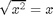•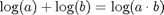•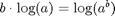•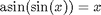Just using simplify to get the result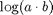on input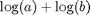does not work:

syms a b
simplify(log(a)+log(b))
ans =
log(a) + log(b)


### Using Assumptions on Variables

Of course, we all know that the ruleapplies only under appropriate mathematical assumptions onand. For example, if we assume thatandare positive, we will get the desired result:

syms a b positive
simplify(log(a)+log(b))
ans =
log(a*b)


To get rid of all previously specified assumptions, use

syms a b clear

### When Things Get More Complicated

Does it mean that setting the right assumptions is a universal solution here? Well, not always!

Assumeandappear as intermediate results in some really huge symbolic computations somewhere in, lets say, line 454 of your MATLAB script. From the context of your application, you know thatandare positive. Now you want MATLAB to automatically compute a simplified form ofin line 455. How will you manage to set the right assumptions?

As an example, think ofandas being something like

syms x y
a = -(x + 1)^(1/2)/((exp(x - y) - sin(x + y)) * ...
(log((x^2 + 1)/(y^2 + 1))/exp(y) + (x - y)^y + ...
1/(x - y)^x));
b = (cos(x)*sin(y))/((x - y)^x*(x + 1)^(1/2)) - ...
(exp(x)*(x - y)^y)/(exp(y)*(x + 1)^(1/2)) + ...
(cos(y)*sin(x))/((x - y)^x*(x + 1)^(1/2)) - ...
(exp(x)*log((x^2 + 1)/(y^2 + 1)))/ ...
(exp(2*y)*(x + 1)^(1/2)) - exp(x)/ ...
(exp(y)*(x - y)^x*(x + 1)^(1/2)) + ...
(cos(x)*sin(y)*(x - y)^y)/(x + 1)^(1/2) + ...
(cos(y)*sin(x)*(x - y)^y)/(x + 1)^(1/2) + ...
(log((x^2 + 1)/(y^2 + 1))*cos(x)*sin(y))/ ...
(exp(y)*(x + 1)^(1/2)) + (log((x^2 + 1)/ ...
(y^2 + 1))*cos(y)*sin(x))/(exp(y)*(x + 1)^(1/2));

Now executing the simplify command does not seem to be helpful:

S = simplify(log(a)+log(b));
pretty(S)

/                                         y
|     sin(x + y)        sin(x + y) (x - y)
log| ------------------- + ------------------- +
|        x        1/2              1/2
\ (x - y)  (x + 1)          (x + 1)

y
#1 sin(x + y)   exp(x) (x - y)
------------- - --------------- -
#2               #2

\
exp(x) #1                  exp(x)           |
------------------- - -------------------------- | +
1/2                 x        1/2 |
exp(2 y) (x + 1)      exp(y) (x - y)  (x + 1)    /

/           1/2    /
log| - ((x + 1)   ) / | (exp(x - y) - sin(x + y))
|                  |
\                  \

/   #1            y      1     \ \ \
| ------ + (x - y)  + -------- | | |
| exp(y)                     x | | |
\                     (x - y)  / / /

where

/  2     \
| x  + 1 |
#1 = log| ------ |
|  2     |
\ y  + 1 /

1/2
#2 = exp(y) (x + 1)


Assumingandto be positive does not significantly improve the result either. The reason is that we need to set such assumptions onandthat would make the expressionsandpositive. We can try to find the right assumptions for this example, but in general it seems like one has to be a genius to guess what's appropriate.

### Using Option IgnoreAnalyticConstraints for Simplification

A possible solution to the problem is to ignore certain analytic constraints, that is, to use the IgnoreAnalyticConstraints option for simplify.

With this option the simplifier internally applies the following rules:

•for all values ofand. In particular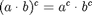for all values of,and.
•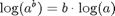for all values ofand. In particular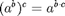for all values of,and.
• If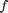andare standard math functions and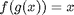holds for all small positive numbers, thenis assumed to be valid for all(for example as in case of).

So how does this work in our example?

simplify(log(a)+log(b),'IgnoreAnalyticConstraints',true)
ans =
0


The result is, because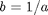. Hence, under the above assumptions, we get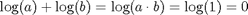.

Of course, it is important to keep in mind that the rules applied by IgnoreAnalyticConstraints are not correct in a strict mathematical sense. Nevertheless, in practice these rules are often very helpful to get simpler results. Another nice side effect is that ignoring some analytic constraints often helps you speed up your computations.

This documentation describes more details on IgnoreAnalyticConstraints.

### Using IgnoreAnalyticConstraints for Equation Solving

Not surprisingly the concept of ignoring analytic constraints also makes sense for equation solving. Imagine that you want to solve the equation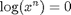for. Ignoring analytic constraints would certainly mean to write this as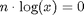. Assumingto be nonzero we get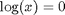and, finally,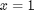.

Without using any restrictions, Symbolic Math Toolbox returns the result:

syms x n
solve(log(x^n),x)
Warning: The solutions are parametrized by the symbols:
k = Z_ intersect Dom::Interval([-1/(2*Re(1/n))],
1/(2*Re(1/n)))

ans =
1/exp((pi*k*2*i)/n)


So you get a parameterized solution which strongly depends on the values of. This is reasonable, because, for example, for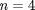you get the four solutions

solve(log(x^4),x)
ans =
1
-1
i
-i


whereas for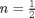there only is one solution:

solve(log(x^(1/2)),x)
ans =
1


Applying IgnoreAnalyticConstraints we get

solve(log(x^n),x,'IgnoreAnalyticConstraints',true)
ans =
1


Also for equations involving roots where no additional symbolic parameters are present, it may be useful to apply IgnoreAnalyticConstraints to get simpler results:

solve(x^(5/2) - 8^(sym(10/3)), 'IgnoreAnalyticConstraints', true)
ans =
16


Here the solver ignores branch cuts during internal simplifications and, hence, returns only one solution.

See the MATLAB doc page on solve for further details.

The IgnoreAnalyticConstraints option can also be used for other Symbolic Math Toolbox functions like the function int for doing symbolic integration. The option is also available for the related MuPAD Notebook Interface functions.

### Have You Tried IgnoreAnalyticConstraints?

Have you tried the IgnoreAnalyticConstraints option to get simpler, shorter, and easier to handle results?

Let me know here.

Published with MATLAB® 7.13

|